Probability 03 Random Variable n n Probability Mass

• Slides: 18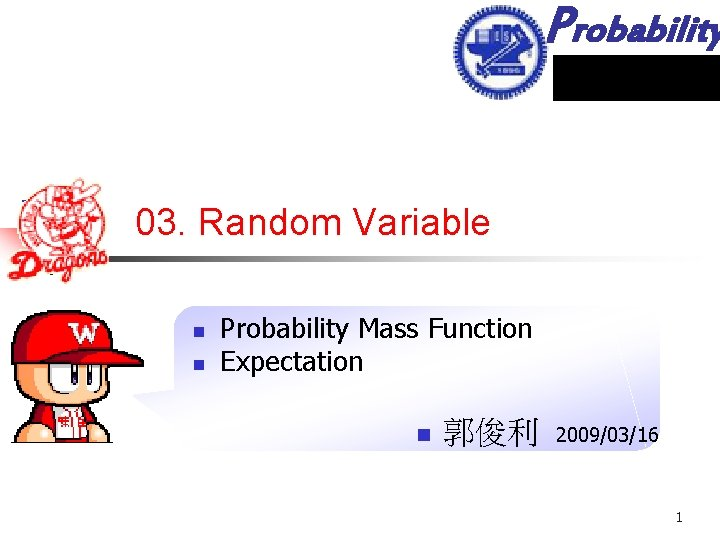Probability 03. Random Variable n n Probability Mass Function Expectation n 郭俊利 2009/03/16 1Outline Probability n Review n n Conditional probability Random variable PMF Conditional PMF 1. 7 ~ 2. 6 2Example 1 Probability n From the set of integers {1, 2, 3, . . . , 100000} a number is selected at random. What is the probability that the sum of its digits is 11? All – (a digit over 11) – (a digit over 10) = H 511 – C 52 2! 3Example 2 Probability n First throw an unbiased die, then throw as many unbiased coins as the point shown on the die. n n What is the probability of obtaining k heads? If 3 heads are obtained, what is the probability that the die showed n? 4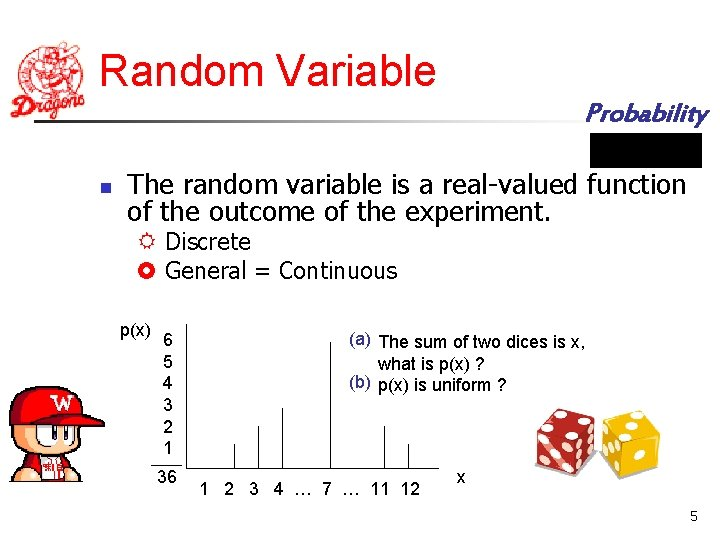Random Variable Probability n The random variable is a real-valued function of the outcome of the experiment. Discrete General = Continuous p(x) 6 5 4 3 2 1 36 (a) The sum of two dices is x, what is p(x) ? (b) p(x) is uniform ? 1 2 3 4 … 7 … 11 12 x 5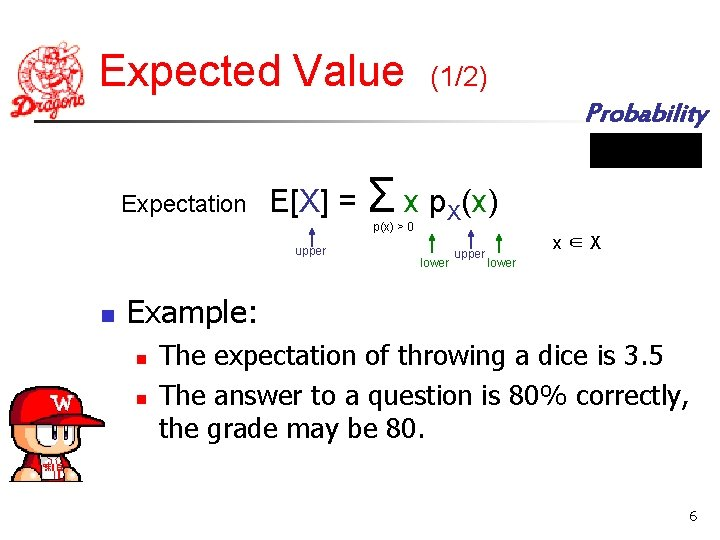Expected Value (1/2) Probability Expectation E[X] = Σ x p. X(x) p(x) > 0 upper n lower upper x∈X lower Example: n n The expectation of throwing a dice is 3. 5 The answer to a question is 80% correctly, the grade may be 80. 6Expected Value (2/2) Probability n E[a] = a E[a. X] = a. E[x] E[a. X + b] = a. E[x] + b n E[g(X)] = n E[X] = n n Σ g(x) px(x) Σ E[Xi] = np (p is uniform!) i=1~n 7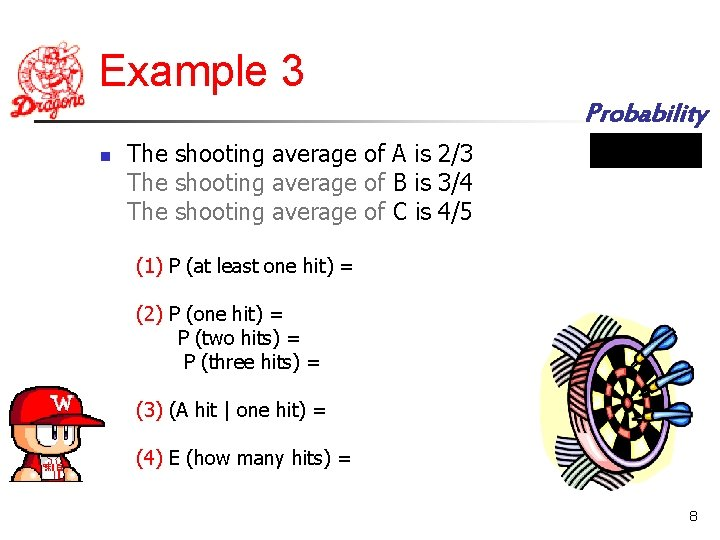Example 3 Probability n The shooting average of A is 2/3 The shooting average of B is 3/4 The shooting average of C is 4/5 (1) P (at least one hit) = (2) P (one hit) = P (two hits) = P (three hits) = (3) (A hit | one hit) = (4) E (how many hits) = 8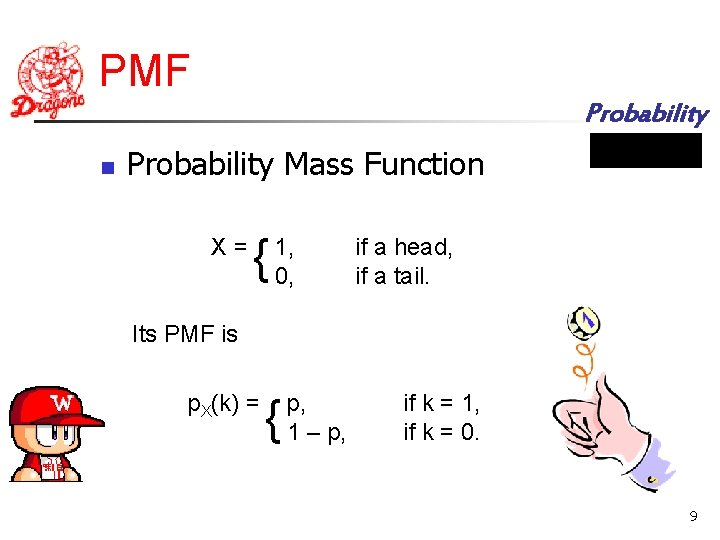PMF Probability n Probability Mass Function X= { 1, 0, if a head, if a tail. Its PMF is p. X(k) = { p, 1 – p, if k = 1, if k = 0. 9Example 4 Probability n Let X be a random variable that takes values from 0 to 9 equal likely. (1) Find the PMF of Y = X mod 3 (2) Find the PMF of Z = 5 mod X+1 n A family has 5 natural children and has adopted 2 girls. Find the PMF of the number of girls out of the 7 children. 10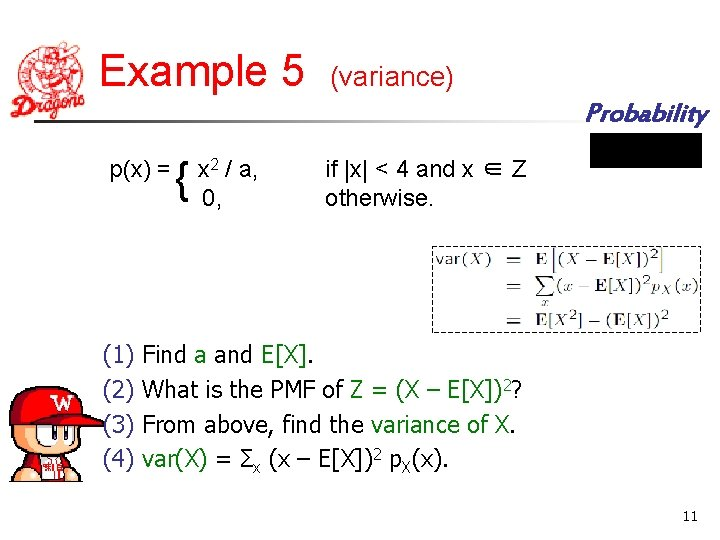Example 5 (variance) Probability p(x) = (1) (2) (3) (4) { x 2 / a, 0, if |x| < 4 and x ∈ Z otherwise. Find a and E[X]. What is the PMF of Z = (X – E[X])2? From above, find the variance of X. var(X) = Σx (x – E[X])2 p. X(x). 11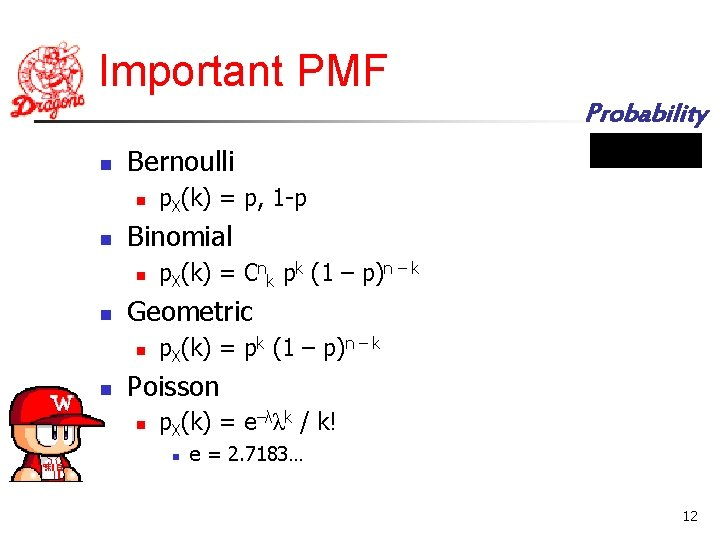Important PMF Probability n Bernoulli n n Binomial n n p. X(k) = Cnk pk (1 – p)n – k Geometric n n p. X(k) = p, 1 -p p. X(k) = pk (1 – p)n – k Poisson n p. X(k) = e–λλk / k! n e = 2. 7183… 12Poisson Probability n Poisson is a good model for Binomial n When p is very small and n is very large, then λ = np n The probability of a wrong words is 0. 1% and there are 1000 words in the document, what is the probability of 5 words found? C 1000 5 0. 0015(0. 999)95 ≒ e– 1 15 5! 13Joint PMF Probability n Joint PMF n n p. X, Y(x, y) = P(X = x, Y = y) Marginal PMF p. X(x) = Σy p. X, Y(x, y) p. Y(y) = Σx p. X, Y(x, y) n Example n p. X, Y(x, y) = 1 / 52 p. X(x) = 13 1 = 1 / 4 52 14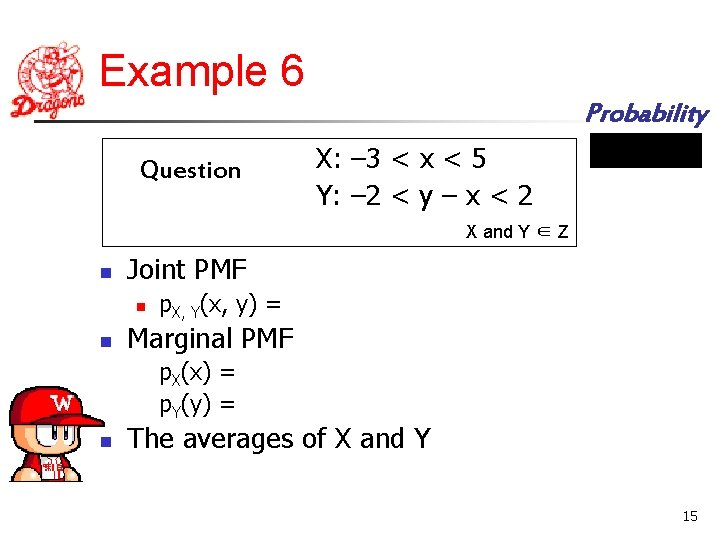Example 6 Probability Question X: – 3 < x < 5 Y: – 2 < y – x < 2 X and Y ∈ Z n Joint PMF n n p. X, Y(x, y) = Marginal PMF p. X(x) = p. Y(y) = n The averages of X and Y 15Example 7 Probability n A perfect coin is tossed n times. Let Yn denote the number of heads obtained minus the number of tails. Find the probability distribution of Yn and its mean. 16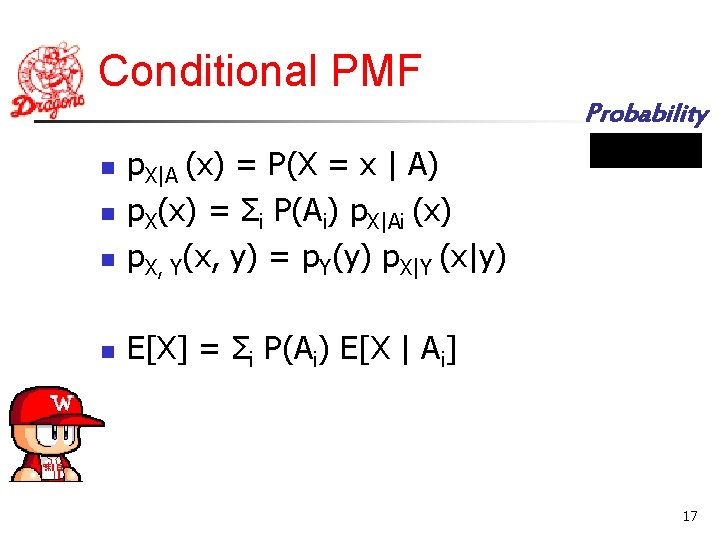Conditional PMF Probability n p. X|A (x) = P(X = x | A) p. X(x) = Σi P(Ai) p. X|Ai (x) p. X, Y(x, y) = p. Y(y) p. X|Y (x|y) n E[X] = Σi P(Ai) E[X | Ai] n n 17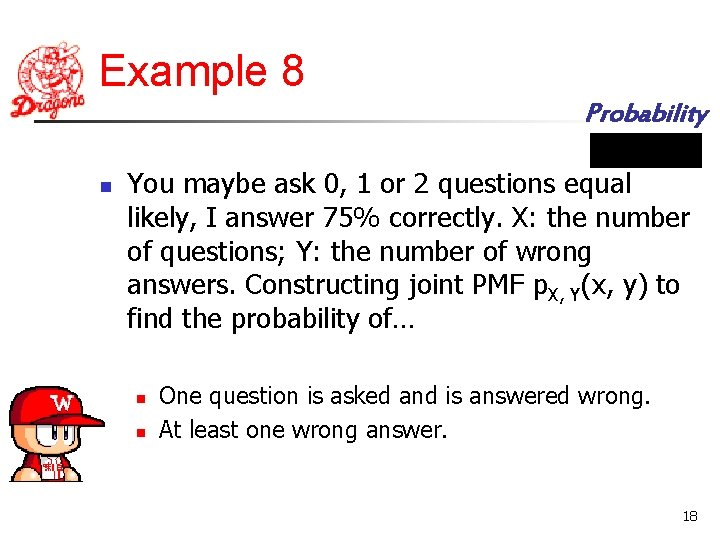Example 8 Probability n You maybe ask 0, 1 or 2 questions equal likely, I answer 75% correctly. X: the number of questions; Y: the number of wrong answers. Constructing joint PMF p. X, Y(x, y) to find the probability of… n n One question is asked and is answered wrong. At least one wrong answer. 18